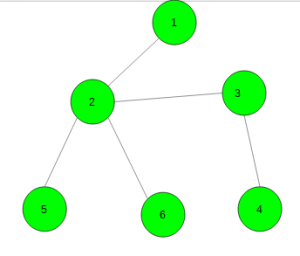Related Articles

# Make a tree with n vertices , d diameter and at most vertex degree k

• Last Updated : 02 Jun, 2021

Given three integers N, D and K. The task is to check whether it is possible to make a tree with exactly N vertices, D diameter (the number of edges in the longest path between any two vertices), and degree of each vertex must be at most K. If it is possible then print all the possible edges otherwise print No.

Examples:

Input: N = 6, D = 3, K = 4
Output:
1 2
2 3
3 4
5 2
6 2Input: N = 6, D = 2, K = 4
Output: N0

Approach: Let’s construct the tree with the following algorithm: If (d > n – 1), print “No” and terminate the program. Otherwise, let’s keep the array deg of the length n which will represent degrees of vertices.
The first step is to construct the diameter of the tree. Let the first (d + 1) vertices form it.

Let’s add d edges to the answer and increase degrees of vertices corresponding to these edges, and if some vertex has degree greater than k, print “No” and terminate the program.

The second (and the last) step is to attach the remaining (n – d – 1) vertices to the tree. Let’s call the vertex free if its degree is less than k. Also, let’s keep all free vertices forming the diameter in some data structure which allows us to take the vertex with the minimum maximal distance to any other vertex and remove such vertices. It can be done by, for example, set of pairs (distv, v), where distv is the maximum distance from the vertex v to any other vertex. Now let’s add all the vertices starting from the vertex (d + 1) (0-indexed) to the vertex n?1, let the current vertex be u. One can get the vertex with the minimum maximal distance to any other vertex, let it be v. Now increase the degree of vertices u and v, add the edge between them, and if v still be free, return it to the data structure, otherwise remove it. The same with the vertex u (it is obvious that its maximal distance to any other vertex will be equal to (distv + 1).

If at any step our data structure will be empty or the minimum maximal distance will equal d, the answer is “No”. Otherwise, we can print the answer.

Below is the implementation of the above approach:

## C++

 `// C++ implementation of the approach``#include ``using` `namespace` `std;` `// Function to Make a tree with n vertices, d as it's``// diameter and degree of each vertex is at most k``void` `Make_tree(``int` `n, ``int` `d, ``int` `k)``{``    ``// If diameter > n - 1``    ``// impossible to build tree``    ``if` `(d > n - 1) {``        ``cout << ``"No"``;``        ``return``;``    ``}` `    ``// To store the degree of each vertex``    ``vector<``int``> deg(n);` `    ``// To store the edge between vertices``    ``vector > ans;` `    ``// To store maximum distance among``    ``// all the paths from a vertex``    ``set > q;` `    ``// Make diameter of tree equals to d``    ``for` `(``int` `i = 0; i < d; ++i) {``        ``++deg[i];``        ``++deg[i + 1];``        ``if` `(deg[i] > k || deg[i + 1] > k) {``            ``cout << ``"NO"` `<< endl;``            ``return``;``        ``}` `        ``// Add an edge between them``        ``ans.push_back(make_pair(i, i + 1));``    ``}` `    ``// Store maximum distance of each vertex``    ``// from other vertices``    ``for` `(``int` `i = 1; i < d; ++i)``        ``q.insert(make_pair(max(i, d - i), i));` `    ``// For next (n - d - 1) edges``    ``for` `(``int` `i = d + 1; i < n; ++i) {` `        ``// If the vertex already has the degree k``        ``while` `(!q.empty() && deg[q.begin()->second] == k)``            ``q.erase(q.begin());` `        ``// If not possible``        ``if` `(q.empty() || q.begin()->first == d) {``            ``cout << ``"No"``;``            ``return``;``        ``}` `        ``// Increase the degree of vertices``        ``++deg[i];``        ``++deg[q.begin()->second];` `        ``// Add an edge between them``        ``ans.push_back(make_pair(i, q.begin()->second));` `        ``// Store the maximum distance of this vertex``        ``// from other vertices``        ``q.insert(make_pair(q.begin()->first + 1, i));``    ``}` `    ``// Print all the edges of the built tree``    ``for` `(``int` `i = 0; i < n - 1; ++i)``        ``cout << ans[i].first + 1 << ``" "``             ``<< ans[i].second + 1 << endl;``}` `// Driver code``int` `main()``{``    ``int` `n = 6, d = 3, k = 4;``    ``Make_tree(n, d, k);` `    ``return` `0;``}`

## Python3

 `# Python3 implementation of the approach` `# Function to Make a tree with n vertices, d as it's``# diameter and degree of each vertex is at most k``def` `Make_tree(n, d, k):``    ` `    ``# If diameter > n - 1``    ``# impossible to build tree``    ``if` `(d > n ``-` `1``):``        ``print``(``"No"``)``        ``return` `    ``# To store the degree of each vertex``    ``deg ``=` `[``0``]``*``(n)` `    ``# To store the edge between vertices``    ``ans ``=` `[]` `    ``# To store maximum distance among``    ``#all the paths from a vertex``    ``q ``=` `{}` `    ``# Make diameter of tree equals to d``    ``for` `i ``in` `range``(d):``        ``deg[i] ``+``=` `1``        ``deg[i ``+` `1``] ``+``=` `1``        ``if` `(deg[i] > k ``or` `deg[i ``+` `1``] > k):``            ``print``(``"NO"``)``            ``return` `        ``# Add an edge between them``        ``ans.append((i, i ``+` `1``))` `    ``# Store maximum distance of each vertex``    ``# from other vertices``    ``for` `i ``in` `range``(``1``, d):``        ``q[(``max``(i, d ``-` `i), i)] ``=` `1` `    ``# For next (n - d - 1) edges``    ``for` `i ``in` `range``(d ``+` `1``, n):``        ``arr ``=` `list``(q.keys())` `        ``# If the vertex already has the degree k``        ``while` `(``len``(q) > ``0` `and` `deg[arr[``0``][``1``]] ``=``=` `k):``            ``del` `q[arr[``0``]]` `        ``# If not possible``        ``if` `(``len``(q) ``=``=` `0` `or` `arr[``0``][``0``] ``=``=` `d):``            ``print` `(``"No"``)``            ``return` `        ``# Increase the degree of vertices``        ``deg[i] ``+``=` `1``        ``deg[arr[``0``][``1``]] ``+``=` `1` `        ``# Add an edge between them``        ``ans.append((i, arr[``0``][``1``]))` `        ``# Store the maximum distance of this vertex``        ``# from other vertices``        ``q[(arr[``0``][``0``] ``+` `1``, i)] ``=` `1` `    ``# Prall the edges of the built tree``    ``for` `i ``in` `range``(n ``-` `1``):``        ``print``(ans[i][``0``] ``+` `1``, ans[i][``1``]``+` `1``)` `# Driver code``if` `__name__ ``=``=` `'__main__'``:``    ``n, d, k ``=` `6``, ``3``, ``4``    ``Make_tree(n, d, k)` `    ``# This code is contributed by mohit kumar 29.`

## Javascript

 ``
Output:
```1 2
2 3
3 4
5 2
6 2```My Personal Notes arrow_drop_up